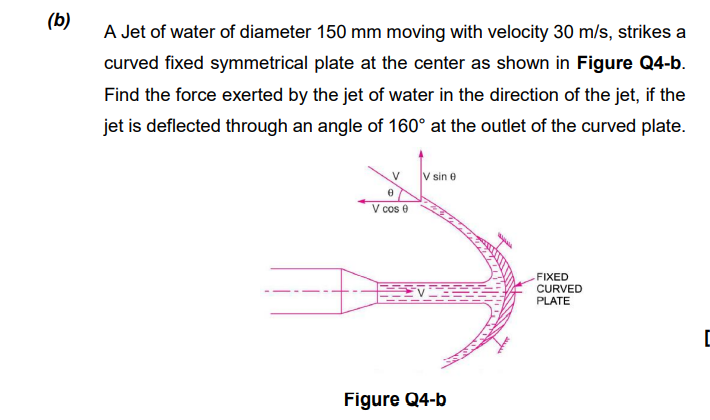# A Jet of water of diameter 150 mm moving with velocity 30 m/s, strikes a curved fixed symmetrical plate at the center as shown in Figure Q4-b. Find the force exerted by the jet of water in the direction of the jet, if the jet is deflected through an angle of 160° at the outlet of the curved plate

Question-AnswerCategory: Fluid MechanicsA Jet of water of diameter 150 mm moving with velocity 30 m/s, strikes a curved fixed symmetrical plate at the center as shown in Figure Q4-b. Find the force exerted by the jet of water in the direction of the jet, if the jet is deflected through an angle of 160° at the outlet of the curved plate

A Jet of water of diameter 150 mm moving with velocity 30 m/s, strikes a curved fixed symmetrical plate at the center as shown in Figure Q4-b. Find the force exerted by the jet of water in the direction of the jet, if the jet is deflected through an angle of 160° at the outlet of the curved plate.